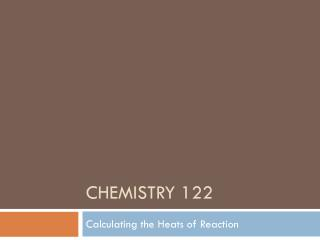DownloadDownload PresentationChemistry 122

# Chemistry 122

Télécharger la présentation## Chemistry 122

- - - - - - - - - - - - - - - - - - - - - - - - - - - E N D - - - - - - - - - - - - - - - - - - - - - - - - - - -

1. ### Chemistry 122

Calculating the Heats of Reaction
2. Hess's Law It is sometimes difficult to measure the change in enthalpy in a reaction because the reaction does not actually happen. It is possible to measure the heat indirectly. Hess's law of heat summation states that if you have two or more thermochemical reactions to give a final equation, then you can also add the heats of reaction to give its final heat of reaction. The law allows us to measure the heat of reaction indirectly.
3. Example One Carbon can exist in the form of a diamond or graphite. Graphite is more stable than diamond but it takes millions of years for the reaction to take place – examining the reaction directly is not practical. Using Hess's law, we can calculate the heat of reaction for turning diamond into graphite – an example of an extremely slow reaction.
4. Thermochemical Equations a. C(s, graphite) + O2(g)→ CO2(g)ΔH = -393.5kJ b. C(s, diamond) + O2(g)→ CO2(g)ΔH = -395.4kJ Write the reverse of equation a: c. CO2(g)→ C(s, graphite) + O2(g)ΔH = +393.5kJ Add it to equation b and cancel out what is found on opposite sides of the arrow: C(s, diamond)→ C(s, graphite)ΔH = -1.9kJ
5. Does this make sense? The conversion of diamond to graphite is an exothermic process, therefore it is a negative enthalpy change. The vice versa holds true for graphite turning to diamond – it is an endothermic process. Diamonds are the world's oldest substances. They are approximately 3.3 million years old (How old is the Earth?). Diamonds are only found deep underground – having been formed by extremely high temperature and pressure reactions.
6. Example Two: We can also use Hess's law when we are examining reactions that produce more products than the one we are interested in. C(s, graphite) + ½ O2(g)→ CO(g)ΔH = ? This reaction is impossible to replicate in the laboratory because carbon dioxide is also produced along with carbon monoxide…
7. More realistic reactions that involve carbon monoxide would look like this: a. C(s, graphite) + O2(g)→ CO2(g)ΔH = -393.5kJ b. CO(g) + ½ O2(g)→ CO2(g)ΔH = -283.0kJ Write the reverse of equation b: CO2(g)→ CO(g) + ½ O2(g) ΔH = +283.0kJ Then eliminate those substances found on both sides of the equation: C(s, graphite) + ½ O2(g)→ CO(g)ΔH = -110.5kJ
8. Making sense of the data The formation of carbon monoxide is exothermic. 110.0kJ of heat is released when it forms from carbon – in graphite form. Compare the two figures on pages 528 – 529. In Figure 17.13, which equation represents decomposition? In Figure 17.14, which is endothermic/exothermic?
9. A simple comparison If you are having trouble seeing the elimination process using Hess's law, consider the following: 1 + 2 = 3 3 + 4 = 5 + 2 If you cancel out the numbers between the two equations that repeat on both sides – what remains?
10. Standard Heats of Formation (ΔHf⁰) The change in enthalpy that accompanies the formation of one mole of a compound from its elements with all substances in their standard states at 25°C and 101.3kPa. Remember what a formation reaction is? Na + Cl2 → NaCl
11. Comparisons of Heats of Formation When looking at a free element, the heat of formation is zero. Diatomic molecules are also set at zero. For a reaction that occurs at standard conditions, you can calculate the heat of reaction by using standard heats of formation. This is referred to as the standard heat of formation (ΔH⁰) It is calculated by finding the difference between all the reactants and products heats of formation in a chemical equation.
12. Therefore, ΔH⁰ = ΔHf⁰ (products) - ΔHf⁰ (reactants)
13. There are two ways to skin a cat. 1. Use Hess's law and add two or more thermochemical equations to give the desired equation. 2. Find the difference between the standard heats of formation for all reactants and the standard heats of formation for all products in the reaction.
14. Figure 17.16, page 532 Copy this figure into your notebook. Make sure you include notes on where each of the enthalpy changes come from. Also, you should write the thermochemical equations for each of the reactions.
15. Questions for practice: Questions 32, 33 – page 531 Also, calculate the standard heat of reaction for the following reaction: 2SO2(g) + O2(g) → 2SO3(g) Questions 34 – 37 – page 532